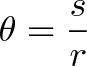## VIDEO: Spiral Sculptures

John Edmark is an artist and professor at Stanford University who has used the Golden Angle to sculpt spirals. The Golden Angle is derived from the Golden Ratio: it is the smaller of the two angles created by dividing the circumference of a circle according to the golden ratio and comes out to be around 137.5°.M x

Degrees and Radians are two different units for measuring angles. Most of us are familiar with degrees as this is the most widely used unit outside of mathematics (or physics).

The degree originates from ancient civilisations who used astronomy to “mark the seasons, predict the future and appease the gods”. The theory of how the degree was conceived is that humans noticed that constellations moved in a full circle every year. Every day, they were off by a small fraction (‘a degree’), and since a day had roughly 360 days, a circle has 360 degrees. Why not 365 degrees you ask? Ancient civilisations used sundials, which weren’t particularly accurate. Additionally, 360 fit nicely into the base-60 number system used by the Babylonians (the main ancient civilisation which used degrees).

Radians is the unit that mathematicians prefer to use. This is due to the fact that whilst degrees measure the angles by how far we “tilt our heads”, radians measure angles by the distance travelled. In order to normalise the angle, you must divide by the radius. Hence,$\displaystyle{Radian = \frac{distance \hspace{10pt} traveled}{radius}}$

or$\displaystyle{\theta = \frac{s}{r}}$

where theta is the angle in radians, arc length is s, and r is radius.

Furthermore, radians are preferred due to the fact that when trigonometric functions are defined in terms of radians they obey simpler relationships between each other. For example, cosine is the derivative of sine, and sin(x) is roughly equal to x for small x. If we defined these functions using degrees, then the derivative of sine would be pi/180 * cosine, and we’d have sin(x) is roughly equal to pi/180 * x for small x.

## Converting between the twoThe general rule when converting from degrees to radians is to multiply the degree by Π /180°. To convert from radians to degrees multiply by 180°/Π.Sources: 1 | 2 | 3

Hope you enjoy, M x